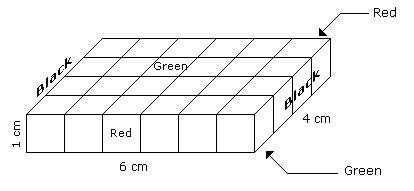# Verbal Reasoning - Cube and Cuboid - Discussion

Discussion Forum : Cube and Cuboid - Cube and Cuboid 1 (Q.No. 2)
Directions to Solve

The following questions are based on the information given below:

1. A cuboid shaped wooden block has 6 cm length, 4 cm breadth and 1 cm height.
2. Two faces measuring 4 cm x 1 cm are coloured in black.
3. Two faces measuring 6 cm x 1 cm are coloured in red.
4. Two faces measuring 6 cm x 4 cm are coloured in green.
5. The block is divided into 6 equal cubes of side 1 cm (from 6 cm side), 4 equal cubes of side 1 cm(from 4 cm side).

2.
How many small cubes will be formed ?
6
12
16
24
Explanation:Number of small cubes = l x b x h = 6 x 4 x 1 = 24

Discussion:
1 comments Page 1 of 1.

Sairarasheed said:   10 years ago
In other cases, we can do volume of cuboid by volume of cube(by calculating the volume).